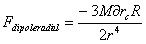## Friday, April 20, 2007

### Radial Dipole Gravity Force in the Equatorial Plane of the Rotation

The radial component of dipole gravity force from the rotating spherical mass in the equatorial plane per unit mass boils down toin the range r>>R, where M is the mass and R the radius of the rotating spherical center and delta rc the relativistic center of mass shift for the corresponding hemisphere.

Because of the latitude angular effect, the force has 1/r^4 dependency instead of 1/r^3 as one may expect from the dipole gravity force.

Simple addition of this force to the Newtonian gravity itself may not be enough to explain the velocity curve, however, it must be noted that the matter distribution in the equatorial plane near and around the center will no longer be like a simple Keplerian orbital distribution. It is expected that there will be a lot more of matter distribution in the long stretch of the equatorial plane starting from the center as shown in the following diagram as the rotational speed gets stronger.Those intermediary matters can not form independent stars because of the excessive gravity forces that prevent them from forming a stable, condensed stellar object.

Dark matters may be just normal matters(baryonic) that can not be visible because they could not be condensed hard and large enough to start the usual stellar thermonuclear fusion process.

As soon as the effect from this fast decreasing 1/r^4 dependent force dies down, in addition to the subsequent reduction of the intermediary matters, the velocity curve will show substantially different path than would be expected from the simple Newtonian gravity.